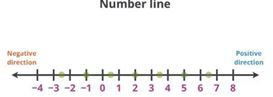# Integers Class 7 Notes: Chapter 1

## Introduction to Integers

### Introduction to Numbers

Natural Numbers : The collection of all the counting numbers is called set of natural numbers. It is denoted by N = {1,2,3,4….}

Whole Numbers: The collection of natural numbers along with zero is called a set of whole numbers. It is denoted by W = { 0, 1, 2, 3, 4, 5, … }## Properties of Addition and Subtraction of Integers

For every integer a and ba+b and ab are integers.

for every integer a and ba+b=b+a

for every integer a,b and c, (a+b)+c=a+(b+c)

For every integer a, a+0=0+a=a here 0 is Additive Identity, since adding 0 to a number leaves it unchanged.
Example : For an integer 2, 2+0 = 0+2 = 2.

For every integer a, a+(a)=0 Here, a is additive inverse of a and a is the additive inverse of-a.
Example : For an integer 2, (– 2) is additive inverse  and for (– 2), additive inverse is 2. [Since + 2 – 2 = 0]

## Properties of Multiplication of Integers

### Properties of Multiplication of Integers

Closure under Multiplication
For every integer a and b, a×b=Integer

Commutative Property of Multiplication
For every integer a and ba×b=b×a

Multiplication by Zero
For every integer a, a×0=0×a=0

Multiplicative Identity
For every integer a, a×1=1×a=a. Here 1 is the multiplicative identity for integers.

Associative property of Multiplication
For every integer a, b  and c,  (a×b)×c=a×(b×c)

Distributive Property of Integers
Under addition and multiplication,  integers show the distributive property.
i.e., For every integer a, b  and ca×(b+c)=a×b+a×c

These properties make calculations easier.

To know more about “Properties of Multiplication of Integers”, visit here.

## Division of Integers

### Division of Integers

When a positive integer is divided by a positive integer, the quotient obtained is a positive integer.
Example: (+6) ÷ (+3) +2

When a negative integer is divided by a negative integer, the quotient obtained is a positive integer.
Example: (-6) ÷ (-3) +2

When a positive integer is divided by a negative integer or negative integer is divided by a positive integer, the quotient obtained is a negative integer.
Example: (-6) ÷ (+3) =2 and Example: (+6) ÷ (-3) 2
To know more about Multiplication and Division of Integers, visit here.

## The Number Line

### Number Line

Representation of integers on a number lineOn a number line when we

(i) add a positive integer for a given integer, we move to the right.
Example : When we add +2 to +3, move 2 places from +3 towards right to get +5

(ii) add a negative integer for a given integer, we move to the left.
Example : When we add -2 to +3, move 2 places from +3 towards left to get +1

(iii) subtract a positive integer from a given integer, we move to the left.
Example: When we subtract +2 from -3, move 2 places from -3 towards left to get -5

(iv) subtract a negative integer from a given integer, we move to the right
Example: When we subtract -2 from -3, move 2 places from -3 towards right to get 1

To know more about Number Lines, visit here.

### Addition and Subtraction of Integers

The absolute value of +7 (a positive integer) is 7
The absolute value of -7 (negative integer) is 7 (its corresponding positive integer)

Addition of two positive integers gives a positive integer.
Example : (+3)+(+4) +7

Addition of two negative integers gives a negative integer.
Example : (3)+(434=7

When one positive and one negative integers are added, we take their difference and place the sign of the bigger integer.
Example : (7)+(25

For subtraction, we add the additive inverse of the integer that is being subtracted, to the other integer.
Example : 56(7356+73 129

## Introduction to Zero

### Integers

Integers are the collection of numbers which is formed by whole numbers and their negatives.
The set of Integers is denoted by Z or I. I =  { …, -4, -3, -2, -1, 0, 1, 2, 3, 4,… }

## Properties of Division of Integers

### Properties of Division of Integers

For every integer a,
(a) a÷0 is not defined
(b) a÷a

Note:  Integers are not closed under division
Example: (– 9) ÷ (– 3) = 2. Result is an integer.
and (3)÷(9)= 1/3. Result is not an integer.

## Multiplication of Integers

### Multiplication of Integers

Product of two positive integers is a positive integer.
Example : (+2)×(+3+6

Product of two negative integers is a positive integer.
Example :(2)×(3+6

Product of a positive and a negative integer is a negative integer.
Example :(+2)×(36 and (2)×(+36

Product of even number of negative integers is positive and product of odd number of negative integers is negative.
These properties make calculations easier.

## Frequently Asked Questions on CBSE Class 7 Maths Notes Chapter 1 Integers

Q1

### What is closure property?

Closure property of whole numbers under addition: The sum of any two whole numbers will always be a whole number, i.e. if a and b are any two whole numbers, a + b will be a whole number.

Q2

### What are the properties of zero?

1. Zero is even 2. Zero is neither positive nor negative 3. Zero is an integer

Q3

### What is the definition of inverse?

A term is said to be in inverse proportion to another term if it increases (or decreases) as the other decreases (or increases). of or relating to an inverse function.